Tamil Nadu Board of Secondary EducationSSLC (English Medium) Class 8th
Advertisement Remove all ads

# Samacheer Kalvi solutions for Mathematics Class 8th Tamil Nadu State Board chapter 5 - Geometry [Latest edition]

Advertisement Remove all ads

#### Chapters## Chapter 5: Geometry

Exercise 5.1Exercise 5.2Exercise 5.3Exercise 5.4Exercise 5.5
Exercise 5.1 [Pages 165 - 166]

### Samacheer Kalvi solutions for Mathematics Class 8th Tamil Nadu State Board Chapter 5 Geometry Exercise 5.1 [Pages 165 - 166]

#### Fill in the blanks with the correct term mention in option.

Exercise 5.1 | Q 1. (i) | Page 165

Corresponding sides of similar triangles are _________

• in proportion

• similar

• corresponding

• congruent

• shape

• area

• equal

Exercise 5.1 | Q 1. (ii) | Page 165

Similar triangles have the same ________ but not necessarily the same size

• in proportion

• similar

• corresponding

• congruent

• shape

• area

• equal

Exercise 5.1 | Q 1. (iii) | Page 165

In any triangle _______ sides are opposite to equal angles

• in proportion

• similar

• corresponding

• congruent

• shape

• area

• equal

Exercise 5.1 | Q 1. (iv) | Page 165

The symbol ≡ is used to represent ________ triangles

• in proportion

• similar

• corresponding

• congruent

• shape

• area

• equal

Exercise 5.1 | Q 1. (v) | Page 165

The symbol ~ is used to represent _________ triangles

• in proportion

• similar

• corresponding

• congruent

• shape

• area

• equal

Exercise 5.1 | Q 2 | Page 165

In the given figure, ∠CIP ≡ ∠COP and ∠HIP ≡ ∠HOP. Prove that IP ≡ OP.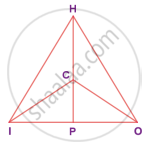Exercise 5.1 | Q 3 | Page 165

In the given figure, AC ≡ AD and ∠CBD ≡ ∠DEC. Prove that ∆BCF ≡ ∆EDF.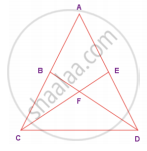Exercise 5.1 | Q 4 | Page 165

In the given figure, ΔBCD is isosceles with base BD and ∠BAE ≡∠DEA. Prove that AB ≡ ED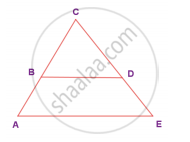Exercise 5.1 | Q 5 | Page 165

In the given figure, D is the midpoint of OE and ∠CDE = 90°. Prove that ΔODC ≡ ΔEDC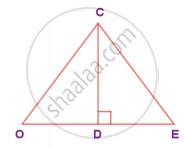Exercise 5.1 | Q 6 | Page 166

Is ΔPRQ ≡ ΔQSP? Why?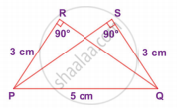Exercise 5.1 | Q 7 | Page 166

From the given figure, prove that ΔABC ~ ΔEDF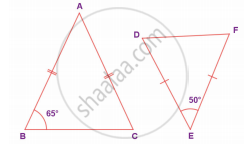Exercise 5.1 | Q 8 | Page 166

In the given figure YH || TE. Prove that ΔWHY ~ ΔWET and also find HE and TE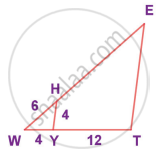Exercise 5.1 | Q 9 | Page 166

In the given figure, if ΔEAT ~ ΔBUN, find the measure of all angles.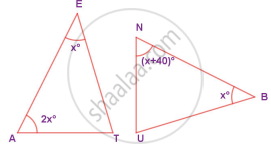Exercise 5.1 | Q 10 | Page 166

In the given figure, UB || AT and CU ≡ CB Prove that ΔCUB ~ ΔCAT and hence ΔCAT is isosceles.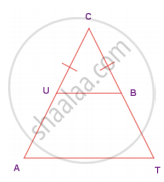#### Objective Type Questions

Exercise 5.1 | Q 11 | Page 166

Two similar triangles will always have ________ angles

• acute

• obtuse

• right

• matching

Exercise 5.1 | Q 12 | Page 166

If in triangles PQR and XYZ, "PQ"/"XY" = "QR"/"ZX" then they will be similar if

• ∠Q = ∠Y

• ∠P = ∠Y

• ∠Q = ∠X

• ∠P = ∠Z

Exercise 5.1 | Q 13 | Page 166

A flag pole 15 m high casts a shadow of 3 m at 10 a.m. The shadow cast by a building at the same time is 18.6 m. The height of the building is

• 90 m

• 91 m

• 92 m

• 93 m

Exercise 5.1 | Q 14 | Page 166

If ∆ABC – ∆PQR in which ∠A = 53° and ∠Q = 77°, then ∠R is

• 50°

• 60°

• 70°

• 80°

Exercise 5.1 | Q 15 | Page 166

In the figure, which of the following statements is true?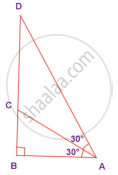• AB = BD

• BD < CD

• AC = CD

• BC = CD

Advertisement Remove all ads
Exercise 5.2 [Pages 177 - 179]

### Samacheer Kalvi solutions for Mathematics Class 8th Tamil Nadu State Board Chapter 5 Geometry Exercise 5.2 [Pages 177 - 179]

#### Fill in the blanks:

Exercise 5.2 | Q 1. (i) | Page 177

If in a ΔPQR, PR2 = PQ2 + QR2, then the right angle of ∆PQR is at the vertex ________

Exercise 5.2 | Q 1. (ii) | Page 177

If ‘l‘ and ‘m’ are the legs and ‘n’ is the hypotenuse of a right angled triangle then, l2 = ________

Exercise 5.2 | Q 1. (iii) | Page 177

If the sides of a triangle are in the ratio 5 : 12 : 13 then, it is ________

Exercise 5.2 | Q 1. (iv) | Page 177

The medians of a triangle cross each other at _______

Exercise 5.2 | Q 1. (v) | Page 177

The centroid of a triangle divides each medians in the ratio _______

#### Say True or False

Exercise 5.2 | Q 2. (i) | Page 178

8, 15, 17 is a Pythagorean triplet

• True

• False

Exercise 5.2 | Q 2. (ii) | Page 178

In a right angled triangle, the hypotenuse is the greatest side

• True

• False

Exercise 5.2 | Q 2. (iii) | Page 178

In any triangle the centroid and the incentre are located inside the triangle

• True

• False

Exercise 5.2 | Q 2. (iv) | Page 178

The centroid, orthocentre, and incentre of a triangle are collinear

• True

• False

Exercise 5.2 | Q 2. (v) | Page 178

The incentre is equidistant from all the vertices of a triangle

• True

• False

Exercise 5.2 | Q 3. (i) | Page 178

Check whether given sides are the sides of right-angled triangles, using Pythagoras theorem

8, 15, 17

Exercise 5.2 | Q 3. (ii) | Page 178

Check whether given sides are the sides of right-angled triangles, using Pythagoras theorem

12, 13, 15

Exercise 5.2 | Q 3. (iii) | Page 178

Check whether given sides are the sides of right-angled triangles, using Pythagoras theorem

30, 40, 50

Exercise 5.2 | Q 3. (iv) | Page 178

Check whether given sides are the sides of right-angled triangles, using Pythagoras theorem

9, 40, 41

Exercise 5.2 | Q 3. (v) | Page 178

Check whether given sides are the sides of right-angled triangles, using Pythagoras theorem

24, 45, 51

Exercise 5.2 | Q 4. (i) | Page 178

Find the unknown side in the following triangles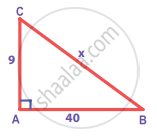Exercise 5.2 | Q 4. (ii) | Page 178

Find the unknown side in the following triangles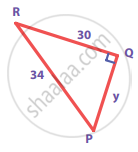Exercise 5.2 | Q 4. (iii) | Page 178

Find the unknown side in the following triangles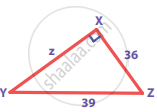Exercise 5.2 | Q 5 | Page 178

An isosceles triangle has equal sides each 13 cm and a base 24 cm in length. Find its height

Exercise 5.2 | Q 6 | Page 178

Find the distance between the helicopter and the ship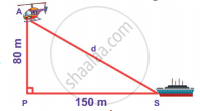Exercise 5.2 | Q 7 | Page 178

In triangle ABC, line I, is a perpendicular bisector of BC.
If BC = 12 cm, SM = 8 cm, find CS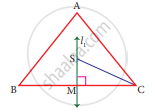Exercise 5.2 | Q 8 | Page 178

Identify the centroid of ∆PQR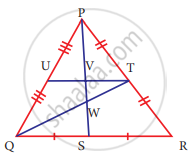Exercise 5.2 | Q 9 | Page 178

Name the orthocentre of ∆PQR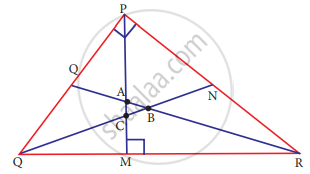Exercise 5.2 | Q 10 | Page 178

In the given figure, A is the midpoint of YZ and G is the centroid of the triangle XYZ. If the length of GA is 3 cm, find XA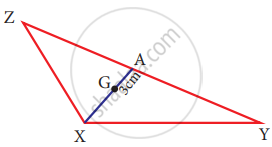Exercise 5.2 | Q 11 | Page 178

If I is the incentre of ∆XYZ, ∠IYZ = 30° and ∠IZY = 40°, find ∠YXZ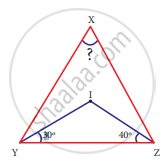#### Objective Type Questions

Exercise 5.2 | Q 12 | Page 179

If ∆GUT is isosceles and right angled, then ∠TUG is ________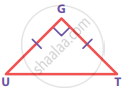• 30°

• 40°

• 45°

• 55°

Exercise 5.2 | Q 13 | Page 179

The hypotenuse of a right angled triangle of sides 12 cm and 16 cm is __________

• 28 cm

• 20 cm

• 24 cm

• 21 cm

Exercise 5.2 | Q 14 | Page 179

The area of a rectangle of length 21 cm and diagonal 29 cm is __________

• 609 cm2

• 580 cm2

• 420 cm2

• 210 cm2

Exercise 5.2 | Q 15 | Page 179

The sides of a right angled triangle are in the ratio 5 : 12 : 13 and its perimeter is 120 units then, the sides are ______________

• 25, 36, 59

• 10, 24, 26

• 36, 39, 45

• 20, 48, 52

Advertisement Remove all ads
Exercise 5.3 [Pages 179 - 180]

### Samacheer Kalvi solutions for Mathematics Class 8th Tamil Nadu State Board Chapter 5 Geometry Exercise 5.3 [Pages 179 - 180]

#### Miscellaneous Practice Problems

Exercise 5.3 | Q 1 | Page 179

In the figure, given that ∠1 = ∠2 and ∠3 ≡ ∠4. Prove that ∆MUG ≡ ∆TUB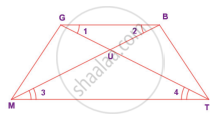Exercise 5.3 | Q 2 | Page 179

From the figure, prove that ∆SUN ~ ∆RAY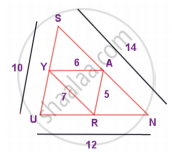Exercise 5.3 | Q 3 | Page 179

The height of a tower is measured by a mirror on the ground at R by which the top of the tower’s reflection is seen. Find the height of the tower. If ∆PQR ~ ∆STR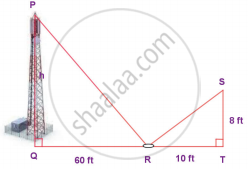Exercise 5.3 | Q 4 | Page 179

Find the length of the support cable required to support the tower with the floor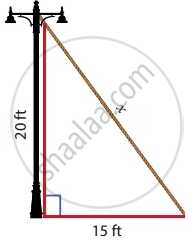Exercise 5.3 | Q 5 | Page 179

Rithika buys an LED TV which has a 25 inches screen. If its height is 7 inches, how wide is the screen? Her TV cabinet is 20 inches wide. Will the TV fit into the cabinet? Give reason

#### Challenging problems

Exercise 5.3 | Q 6 | Page 180

In the figure, ∠TMA ≡∠IAM and ∠TAM ≡ ∠IMA. P is the midpoint of MI and N is the midpoint of AI. Prove that ΔPIN ~ ΔATM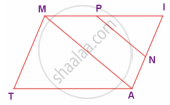Exercise 5.3 | Q 7 | Page 180

In the figure, if ∠FEG ≡ ∠1 then, prove that DG2 = DE.DF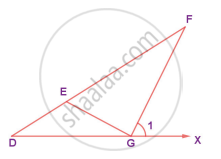Exercise 5.3 | Q 8 | Page 180

The diagonals of the rhombus is 12 cm and 16 cm. Find its perimeter. (Hint: the diagonals of rhombus bisect each other at right angles)

Exercise 5.3 | Q 9 | Page 180

In the figure, find AR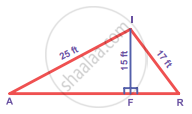Exercise 5.3 | Q 10. (i) | Page 180

In ∆DEF, DN, EO, FM are medians and point P is the centroid. Find the following

If DE = 44, then DM = ?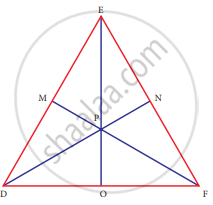Exercise 5.3 | Q 10. (ii) | Page 180

In ∆DEF, DN, EO, FM are medians and point P is the centroid. Find the following

If PD = 12, then PN = ?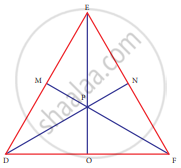Exercise 5.3 | Q 10. (iii) | Page 180

In ∆DEF, DN, EO, FM are medians and point P is the centroid. Find the following

If DO = 8, then FD = ?Exercise 5.3 | Q 10. (iv) | Page 180

In ∆DEF, DN, EO, FM are medians and point P is the centroid. Find the following

If OE = 36 then EP = ?Advertisement Remove all ads
Exercise 5.4 [Page 192]

### Samacheer Kalvi solutions for Mathematics Class 8th Tamil Nadu State Board Chapter 5 Geometry Exercise 5.4 [Page 192]

Exercise 5.4 | Q I. 1. | Page 192

Construct the following quadrilaterals with the given measurements and also find their area.

ABCD, AB = 5 cm, BC = 4.5 cm, CD = 3.8 cm, DA = 4.4 cm and AC = 6.2 cm

Exercise 5.4 | Q I. 2. | Page 192

Construct the following quadrilaterals with the given measurements and also find their area.

PLAY, PL = 7 cm, LA = 6 cm, AY = 6 cm, PA = 8 cm and LY = 7 cm

Exercise 5.4 | Q I. 3. | Page 192

Construct the following quadrilaterals with the given measurements and also find their area.

PQRS, PQ = QR = 3.5 cm, RS = 5.2 cm, SP = 5.3 cm and ∠Q = 120°

Exercise 5.4 | Q I. 4. | Page 192

Construct the following quadrilaterals with the given measurements and also find their area.

MIND, MI = 3.6 cm, ND = 4 cm, MD = 4 cm, ∠M = 50° and ∠D = 100°

Exercise 5.4 | Q I. 5. | Page 192

Construct the following quadrilaterals with the given measurements and also find their area.

AGRI, AG = 4.5 cm, GR = 3.8 cm, ∠A = 60°, ∠G = 110° and ∠R = 90°

Exercise 5.4 | Q II. 1. | Page 192

Construct the following trapeziums with the given measures and also find their area.

AIMS with bar("AI") || bar("SM"), = 6 cm, IM = 5 cm, AM = 9 cm and MS = 6.5 cm

Exercise 5.4 | Q II. 2. | Page 192

Construct the following trapeziums with the given measures and also find their area.

CUTE with bar("CU") || bar("ET"), CU = 7 cm, ∠UCE = 80° CE = 6 cm and TE = 5 cm

Exercise 5.4 | Q III. 3. | Page 192

Construct the following trapeziums with the given measures and also find their area.

ARMY with bar("AR") || bar("YM"), AR = 7 cm, RM = 6.5 cm ∠RAY = 100° and ∠ARM = 60°

Exercise 5.4 | Q II. 4. | Page 192

Construct the following trapeziums with the given measures and also find their area.

CITY with bar("CI") || bar("YT"), CI = 7 cm, IT = 5.5 cm, TY = 4 cm and YC = 6 cm

Advertisement Remove all ads
Exercise 5.5 [Page 209]

### Samacheer Kalvi solutions for Mathematics Class 8th Tamil Nadu State Board Chapter 5 Geometry Exercise 5.5 [Page 209]

Exercise 5.5 | Q I. 1. | Page 209

Construct the following parallelograms with the given measurements and find their area.

ARTS, AR = 6 cm, RT = 5 cm and ∠ART = 70°

Exercise 5.5 | Q I. 2. | Page 209

Construct the following parallelograms with the given measurements and find their area.

CAMP, CA = 6 cm, AP = 8 cm and CP = 5.5 cm

Exercise 5.5 | Q I. 3. | Page 209

Construct the following parallelograms with the given measurements and find their area.

EARN, ER = 10 cm, AN = 7 cm and ∠EOA = 110° where bar("ER") and bar("AN") intersect at O

Exercise 5.5 | Q I. 4. | Page 209

Construct the following parallelograms with the given measurements and find their area.

GAIN, GA = 7.5 cm, GI = 9 cm and ∠GAI = 100°

Exercise 5.5 | Q II. (i) | Page 209

Construct the following rhombuses with the given measurements and also find their area.

FACE, FA = 6 cm and FC = 8 cm

Exercise 5.5 | Q II. (ii) | Page 209

Construct the following rhombuses with the given measurements and also find their area.

CAKE, CA = 5 cm and ∠A = 65°

Exercise 5.5 | Q II. (iii) | Page 209

Construct the following rhombuses with the given measurements and also find their area.

LUCK, LC = 7.8 cm and UK = 6 cm

Exercise 5.5 | Q II. (iv) | Page 209

Construct the following rhombuses with the given measurements and also find their area.

PARK, PR = 9 cm and ∠P = 70°

Exercise 5.5 | Q III. (i) | Page 209

Construct the following rectangles with the given measurements and also find their area.

HAND, HA = 7 cm and AN = 4 cm

Exercise 5.5 | Q III. (ii) | Page 209

Construct the following rectangles with the given measurements and also find their area.

LAND, LA = 8 cm and AD = 10 cm

Exercise 5.5 | Q IV. (i) | Page 209

Construct the following squares with the given measurements and also find their area.

EAST, EA = 6.5 cm

Exercise 5.5 | Q IV. (ii) | Page 209

Construct the following squares with the given measurements and also find their area.

WEST, WS = 7.5 cm

Advertisement Remove all ads

## Chapter 5: Geometry

Exercise 5.1Exercise 5.2Exercise 5.3Exercise 5.4Exercise 5.5## Samacheer Kalvi solutions for Mathematics Class 8th Tamil Nadu State Board chapter 5 - Geometry

Samacheer Kalvi solutions for Mathematics Class 8th Tamil Nadu State Board chapter 5 (Geometry) include all questions with solution and detail explanation. This will clear students doubts about any question and improve application skills while preparing for board exams. The detailed, step-by-step solutions will help you understand the concepts better and clear your confusions, if any. Shaalaa.com has the Tamil Nadu Board of Secondary Education Mathematics Class 8th Tamil Nadu State Board solutions in a manner that help students grasp basic concepts better and faster.

Further, we at Shaalaa.com provide such solutions so that students can prepare for written exams. Samacheer Kalvi textbook solutions can be a core help for self-study and acts as a perfect self-help guidance for students.

Concepts covered in Mathematics Class 8th Tamil Nadu State Board chapter 5 Geometry are Constructing a Quadrilateral When Two Diagonals and Three Sides Are Given, Construct a Quadrilateral When Its Four Sides and One Angle Are Given., Congruency of Shapes, Similar Figures, Congruence of Triangles, Criteria for Congruence of Triangles, SSS Congruence Criterion, SAS Congruence Criterion, ASA Congruence Criterion, RHS Congruence Criterion, Similarity of Triangles, The Property of the Angle Bisectors of a Triangle, Converse of Pythagoras Theorem, Point of Concurrency, Median of a Triangle, Altitudes of a Triangle, Constructing a Quadrilateral When the Lengths of Four Sides and a Diagonal Are Given, Perpendicular Bisectors of a Triangle, Construction of a Rhombus When One Side and One Angle Are Given., Construction of a Rhombus When Two Diagonals Are Given., Circumcentre of a Triangle, Right-angled Triangles and Pythagoras Property, Constructing a Trapezium When Its Two Sides and Two Angles Are Given., Constructing a Trapezium When Its Four Sides Are Given., Constructing a Parallelogram When Its Two Adjacent Sides and One Angle Are Given., Constructing a Parallelogram When Its Two Adjacent Sides and One Diagonal Are Given., Constructing a Parallelogram When Its Two Diagonals and One Included Angle Are Given., Constructing a Parallelogram When Its One Side, One Diagonal and One Angle Are Given., Construction of a Rhombus When One Side and One Diagonal Are Given., Construction of a Rhombus When One Diagonal and One Angle Are Given., Construction of a Rectangle When Its Length and Breadth Are Given., Construction of a Rectangle When a Side and a Diagonal Are Given., Constructing a Quadrilateral When Three Sides and Two Included Angles Are Given, Constructing a Quadrilateral When Two Adjacent Sides and Three Angles Are Known, Constructing a trapezium when its three sides and one diagonal are given, Constructing a Trapezium When Its Three Sides and One Angle Are Given, Construction of a Square When Its Side is Given., Construction of a Square When Its Diagonal is Given..

Using Samacheer Kalvi Class 8th solutions Geometry exercise by students are an easy way to prepare for the exams, as they involve solutions arranged chapter-wise also page wise. The questions involved in Samacheer Kalvi Solutions are important questions that can be asked in the final exam. Maximum students of Tamil Nadu Board of Secondary Education Class 8th prefer Samacheer Kalvi Textbook Solutions to score more in exam.

Get the free view of chapter 5 Geometry Class 8th extra questions for Mathematics Class 8th Tamil Nadu State Board and can use Shaalaa.com to keep it handy for your exam preparation

Advertisement Remove all ads
Share
Notifications

View all notifications

Forgot password?